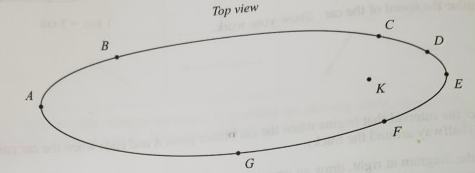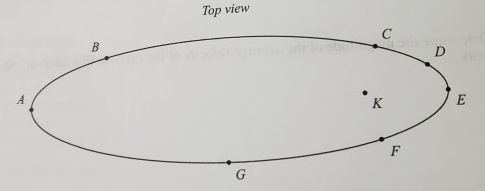# Problem: An object moves clockwise along the trajectory below (top view solution). The acceleration varies but is always directed toward point K.a. On the diagram below, indicate the direction of the acceleration at each of the points A-G.b. For each of the points A-G, state whether the object is speeding up, slowing down, or neither.c. On the diagram below, draw a velocity vector for each of the points A-G. The relative magnitudes of these vectors should be qualitatively correct.

###### FREE Expert Solution

a.

The acceleration at all the points will be acting towards point K.

Therefore, the direction of the acceleration vector is shown in the figure below:

89% (115 ratings)###### Problem Details

An object moves clockwise along the trajectory below (top view solution). The acceleration varies but is always directed toward point K.

a. On the diagram below, indicate the direction of the acceleration at each of the points A-G.b. For each of the points A-G, state whether the object is speeding up, slowing down, or neither.

c. On the diagram below, draw a velocity vector for each of the points A-G. The relative magnitudes of these vectors should be qualitatively correct.